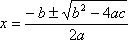Don't have an account? Register now to chat, post, use our tools, and much more.
So I am making a quadratic solver program to help out in my math class, but I have run into a problem.
(-B+√(B²4AC))/(2A)
This equation will correctly find the roots of a quadratic equation when entered on the home screen (A, B, and C are all stored values). However, the results of the same equation with the same values are different when read by the program interpreter. Does anybody know why or how to get around this?
The formula should be interpreted in exactly the same way in a program as on the home screen. Can you please put your code here so we can see if there is a problem? Don't forget that you also need the "-sqrt(..." part.
I don't have my link cable right now but I can post the code later at some point. What is the "-sqrt(..." part? Is that some obscure command or something?
No, the solutions of the equation are both (-B+sqrt(B^2-4AC))/(2A) and (-B-sqrt(B^2-4AC))/(2A). I don't think that is the error though.
Kydapoot wrote:
I don't have my link cable right now but I can post the code later at some point. What is the "-sqrt(..." part? Is that some obscure command or something?Oh, you meant square root. And yes, I have both versions of the equation.
-sqrt( is the minus part of the plus or minus the square root of b squared minus 4ac
(-B+√(B²4AC))/(2A)→E
and
(-B√(B²4AC))/(2A)→F
are my equations.
What are the different answers that you get in the program and on the home screen?
Now its acting up differently. It works correctly most of the time, but when A is positive, B is O, and C is positive it gives error data type upon storing into E through the equation.
Kydapoot wrote:
Now its acting up differently. It works correctly most of the time, but when A is positive, B is O, and C is positive it gives error data type upon storing into E through the equation.

That is probably because the result is complex in that case. I'm not exactly sure why it would throw an Error: Data Type since the real variables can also be complex variables, but it might have something to do with how you are processing the result. What do you mean by "storing into E through the equation" exactly?

Try this and see if it works:

Code:
```Prompt A,B,C sqrt(B²-4AC ({Ans,~Ans}-B)/(2A ```
It worked! I don't know why it was acting up before but my calc has always been a bit buggy.
By storing E I meant I plugged a b and c into the quadratic formula and stored the result to e, but that's irrelevant now anyway.
Did it start working without you changing a single piece of code? That sounds like very strange behavior.
Upon further testing, it is actually not working. Jonbush's solution worked for a couple of simple ones but solved most equations incorrectly.
See what happens when you set the calculator to complex mode (a+bi).

Register to Join the Conversation
Have your own thoughts to add to this or any other topic? Want to ask a question, offer a suggestion, share your own programs and projects, upload a file to the file archives, get help with calculator and computer programming, or simply chat with like-minded coders and tech and calculator enthusiasts via the site-wide AJAX SAX widget? Registration for a free Cemetech account only takes a minute.

»
» All times are GMT - 5 Hours

You cannot post new topics in this forum
You cannot reply to topics in this forum
You cannot edit your posts in this forum
You cannot delete your posts in this forum
You cannot vote in polls in this forum